Updating search results...

# 30 Results

View
Selected filters:
• NC.Math.8.G.8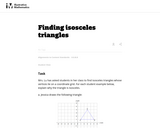Unrestricted Use
CC BY
Rating
0.0 stars

This is a task from the Illustrative Mathematics website that is one part of a complete illustration of the standard to which it is aligned. Each task has at least one solution and some commentary that addresses important asects of the task and its potential use. Here are the first few lines of the commentary for this task: Mrs. Lu has asked students in her class to find isosceles triangles whose vertices lie on a coordinate grid. For each student example below, explain wh...

Subject:
Mathematics
Material Type:
Activity/Lab
Provider:
Illustrative Mathematics
Provider Set:
Illustrative Mathematics
Author:
Illustrative Mathematics
08/05/2013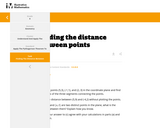Unrestricted Use
CC BY
Rating
0.0 stars

This is a task from the Illustrative Mathematics website that is one part of a complete illustration of the standard to which it is aligned. Each task has at least one solution and some commentary that addresses important aspects of the task and its potential use.

Subject:
Mathematics
Material Type:
Activity/Lab
Provider:
Illustrative Mathematics
Provider Set:
Illustrative Mathematics
Author:
Illustrative Mathematics
05/03/2019Conditional Remix & Share Permitted
CC BY-NC-SA
Rating
0.0 stars

This 8th grade Math&nbsp;parent guide&nbsp;explains the content in straightforward terms so they can support their children&rsquo;s learning at home and will&nbsp;encourage&nbsp;caretaker engagement&nbsp;with lessons.

Subject:
Mathematics
Material Type:
Reference Material
Author:
Kelly Rawlston
Letoria Lewis
01/10/2022Conditional Remix & Share Permitted
CC BY-NC-SA
Rating
0.0 stars

Our Teacher Guides are meant to support the use of our online course and unit content. Please use these to accompany the use of our content and for ideas to support struggling learners, those needing extension and for additional resources.

Subject:
Mathematics
Material Type:
Curriculum
Teaching/Learning Strategy
Author:
Kelly Rawlston
Letoria Lewis
05/16/2022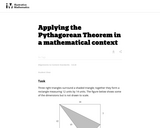Unrestricted Use
CC BY
Rating
0.0 stars

This task requires students to apply the Pythagorean Theorem.

Subject:
Mathematics
Material Type:
Activity/Lab
Provider:
Illustrative Mathematics
Provider Set:
Illustrative Mathematics
Author:
Illustrative Mathematics
05/01/2012Rating
0.0 stars

Students learn how to use the Pythagorean theorem and are introduced to an important application of it.

Subject:
Mathematics
Material Type:
Activity/Lab
Provider:
University of Kentucky
Author:
Julie Gibbs and Tara Bennett
02/26/2019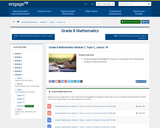Conditional Remix & Share Permitted
CC BY-NC-SA
Rating
0.0 stars

For this lesson, students apply the Pythagorean Theorem to real world and mathematical problems in two dimensions.

Subject:
Mathematics
Material Type:
Lesson Plan
Provider:
EngageNY
02/24/2017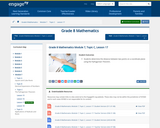Conditional Remix & Share Permitted
CC BY-NC-SA
Rating
0.0 stars

For this lesson, students determine the distance between two points on a coordinate plane using the Pythagorean Theorem.

Subject:
Mathematics
Material Type:
Lesson Plan
Provider:
EngageNY
02/24/2017Rating
0.0 stars

Students use dot grids and the Pythagorean Theorem to find the distance between two points. They also elate areas of squares to the lengths of sides. (See pages 20-22)

Provider:
Mathematics Department At Buffalo State College
Author:
math.buffalostate.edu
06/24/2019Conditional Remix & Share Permitted
CC BY-NC-SA
Rating
0.0 stars

Site a water tower shared by two towns at the midpoint and determine the costs involved using the Pythagorean theorem.

Subject:
Mathematics
Material Type:
Activity/Lab
Author:
ESRI
04/11/2020Educational Use
Rating
0.0 stars

Students use simple materials to design an open spectrograph so they can calculate the angle light is bent when it passes through a holographic diffraction grating. A holographic diffraction grating acts like a prism, showing the visual components of light. After finding the desired angles, students use what they have learned to design their own spectrograph enclosure.

Subject:
Applied Science
Engineering
Material Type:
Activity/Lab
Provider:
TeachEngineering
Provider Set:
TeachEngineering
10/14/2015Conditional Remix & Share Permitted
CC BY-NC
Rating
0.0 stars

Students will use distance and perimeter on the coordinate plane to determine which fence company offers the best price for fencing a yard. Depending on the level of the class, students can use the Pythagorean Theorem or the distance formula.

Subject:
Mathematics
Material Type:
Activity/Lab
Assessment
Formative Assessment
Homework/Assignment
07/10/2020Conditional Remix & Share Permitted
CC BY-NC
Rating
0.0 stars

Students will use distance and perimeter on the coordinate plane to determine which fence company offers the best price for fencing a yard. Depending on the level of the class, students can use the Pythagorean Theorem or the distance formula.

Subject:
Mathematics
Material Type:
Activity/Lab
Assessment
Formative Assessment
Homework/Assignment
06/08/2020Conditional Remix & Share Permitted
CC BY-NC
Rating
0.0 stars

Students will use distance and perimeter on the coordinate plane to determine which fence company offers the best price for fencing a yard. Depending on the level of the class, students can use the Pythagorean Theorem or the distance formula.

Subject:
Mathematics
Material Type:
Activity/Lab
Assessment
Formative Assessment
Homework/Assignment
11/06/2019Conditional Remix & Share Permitted
CC BY-NC
Rating
0.0 stars

Students will use distance and perimeter on the coordinate place to determine which fence company offers the best price for fencing a yard. Depending on the level of the class, students can use the Pythagorean Theorem or the distance formula.

Subject:
Mathematics
Material Type:
Activity/Lab
Assessment
Formative Assessment
Homework/Assignment
11/06/2019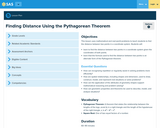Educational Use
Rating
0.0 stars

This lesson uses mathematical and real-world problems to teach students to find the distance between two points in a coordinate system. Students will: learn to find the distance between two points in a coordinate system given the coordinates of both points; learn that the formula used to find the distance between two points is an alternate form of the Pythagorean theorem.

Subject:
Mathematics
Material Type:
Lesson Plan
Provider:
Commonwealth of Pennsylvania
Author:
Commonwealth of Pennsylvania
04/23/2019Educational Use
Rating
0.0 stars

This video demonstrates how to find the distance between two points with the Pythagorean theorem.

Subject:
Mathematics
Material Type:
Interactive
Provider:
Author:
04/23/2019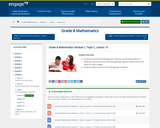Conditional Remix & Share Permitted
CC BY-NC-SA
Rating
0.0 stars

For this lesson, students know that the Pythagorean Theorem can be interpreted as a statement about the areas of similar geometric figures constructed on the sides of a right triangle. Students explain a proof of the Pythagorean Theorem.

Subject:
Mathematics
Material Type:
Lesson Plan
Provider:
EngageNY
02/24/2017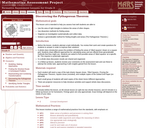Only Sharing Permitted
CC BY-NC-ND
Rating
0.0 stars

This lesson unit is intended to help teachers assess how well students are able to: use the area of right triangles to deduce the areas of other shapes; use dissection methods for finding areas; organize an investigation systematically and collect data; deduce a generalizable method for finding lengths and areas (The Pythagorean Theorem.)

Subject:
Geometry
Mathematics
Material Type:
Assessment
Lesson Plan
Provider:
Shell Center for Mathematical Education
Provider Set:
Mathematics Assessment Project (MAP)
06/24/2019Unrestricted Use
Public Domain
Rating
0.0 stars

In this unit students will:-Understand Pythagorean Theorem-Use Converse of&nbsp;Pythagorean Theorem to prove if a triangle is a right triange-Use Pythagorean Theorem to find distance between two points on a coordinate plane-Use Pythagorean Theorem to solve real world problem situations

Subject:
Mathematics
Material Type:
Unit of Study
Author:
Lindsey Fodrie